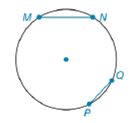Chapter 6.4, Problem 21EElementary Geometry For College St...

7th Edition
Alexander + 2 others
ISBN: 9781337614085

Solutions

Chapter
SectionElementary Geometry For College St...

7th Edition
Alexander + 2 others
ISBN: 9781337614085
Textbook Problem

a) If M N > P Q , write an inequality that compares the measures of minor arcs M N ⌢ and P Q ⌢ .b) If M N > P Q , write and inequality that compares the measures of major arcs M P N ⌢ and P M Q ⌢ .To determine

(a)

To write:

An inequality that compares the measures of minor arcs MN and PQ.

Explanation

Given:

MN>PQ and the figure given below,

Theorem Used:

In a circle (or in congruent circles) containing two unequal chords, the longer chord corresponds to the greater minor arc.

Calculation:

Using following theorem:

Since the chords are unequal i.e. MN>PQ and from the given figure,

To determine

(b)

To write:

An inequality that compares the measures of major arcs MPN and PMQ.

Still sussing out bartleby?

Check out a sample textbook solution.

See a sample solution

The Solution to Your Study Problems

Bartleby provides explanations to thousands of textbook problems written by our experts, many with advanced degrees!

Get Started

A sample of n = 9 scores has X = 108. What is the sample mean?

Essentials of Statistics for The Behavioral Sciences (MindTap Course List)

In Exercises 19-24, find the functions f + g, f g, fg, and fg. 20. f(x) = x1; g(x) = x3 + 1

Applied Calculus for the Managerial, Life, and Social Sciences: A Brief Approach

True or False: f(x) = x2 is decreasing for 10 x 1.

Study Guide for Stewart's Single Variable Calculus: Early Transcendentals, 8th

True or False: converges.

Study Guide for Stewart's Multivariable Calculus, 8th